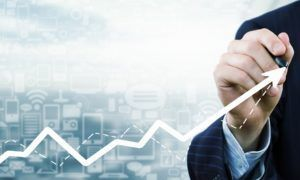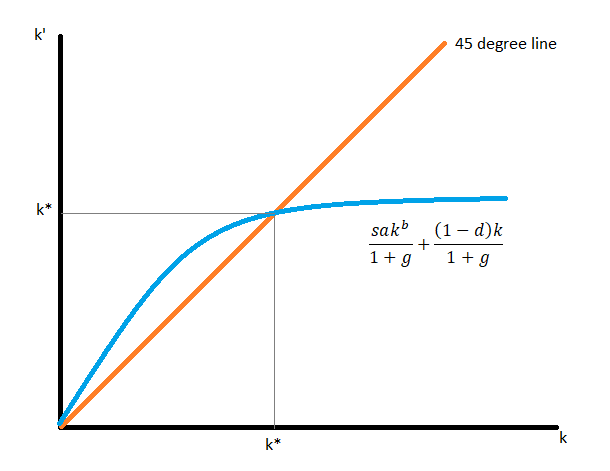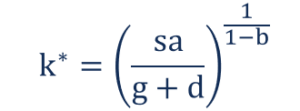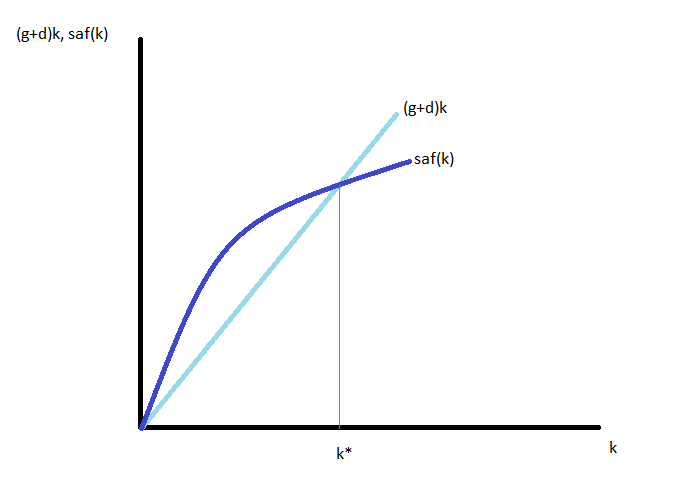Solow Growth Model

An exogenous model of economic growth that analyzes changes in economic output over time

What is the Solow Growth Model?

The Solow Growth Model is an exogenous model of economic growth that analyzes changes in the level of output in an economy over time as a result of changes in the population growth rate, the savings rate, and the rate of technological progress.The Solow Growth Model, developed by Nobel Prize-winning economist Robert Solow, was the first neoclassical growth model and was built upon the Keynesian Harrod-Domar model. The Solow model is the basis for the modern theory of economic growth.

Simplified Representation of the Solow Growth Model

Below is a simplified representation of the Solow Model.

Assumptions:

1. The population grows at a constant rate g. Therefore, current population (represented by N) and future population (represented by N’) are linked through the population growth equation N’ = N(1+g). If the current population is 100 and the growth rate of population is 2%, the future population is 102.
2. All consumers in the economy save a constant proportion ‘s’ of their incomes and consume the rest. Therefore, consumption (represented by C) and output (represented by Y) are linked through the consumption equation C= (1+s)Y. If a consumer earns 100 units of output as income and the savings rate is 40%, then the consumer consumes 60 units and saves 40 units.
3. All firms in the economy produce output using the same production technology that takes in capital and labor as inputs. Therefore, the level of output (represented by Y), the level of capital (represented by K), and the level of labor (represented by L) are all linked through the production function equation Y = aF(K,L).

The Solow Growth Model assumes that the production function exhibits constant-returns-to-scale (CRS). Under such an assumption, if we double the level of capital stock and double the level of labor, we exactly double the level of output. As a result, much of the mathematical analysis of the Solow model focuses on output per worker and capital per worker instead of aggregate output and aggregate capital stock.

1. Present capital stock (represented by K), future capital stock (represented by K’), the rate of capital depreciation (represented by d), and level of capital investment (represented by I) are linked through the capital accumulation equation K’= K(1-d) + I.

Solving the Solow Growth Model

1. In our analysis, we assume that the production function takes the following form: Y = aKbL1-b  where 0 < b < 1. The production function is known as the Cobb-Douglas Production function, which is the most widely used neoclassical production function. Together with the assumption that firms are competitive, i.e., they are price-taking firms, the coefficient b is the capital share (the share of income that capital receives).
1. Therefore, output per worker is given through the following equation: y = akb where y = Y/L (output per worker and k = K/L (capital stock per worker)
2. Under the assumption of competitive equilibrium, we get the following:
• The income-expenditure identity holds as an equilibrium condition: Y = C + I
• Consumer’s budget constraint: Y = C + S
• Therefore, in equilibrium: I = S = sY.
• The capital accumulation equation becomes: K’ = (1–d)K + sY
1. The capital accumulation equation in per worker times is given through the following equation: (1 + g)k’ = (1 – d)k + sy = (1 – d)k + saf(k) = (1 – d)k + sakb
2. The solution concept used is that of a steady state. The steady state is a state where the level of capital per worker does not change. Consider the graph below:1. The steady state is found by solving the following equation: k’ = k => (1 + g)k = (1 – d)k + sakb
2. Therefore, the steady state value of capital per worker and the steady state value of output per worker are the following:Implications of the Solow Growth Model

There is no growth in the long term. If countries have the same g (population growth rate), s (savings rate), and d (capital depreciation rate), then they have the same steady state, so they will converge, i.e., the Solow Growth Model predicts conditional convergence. Along this convergence path, a poorer country grows faster.

Countries with different saving rates have different steady states, and they will not converge, i.e. the Solow Growth Model does not predict absolute convergence. When saving rates are different, growth is not always higher in a country with lower initial capital stock.